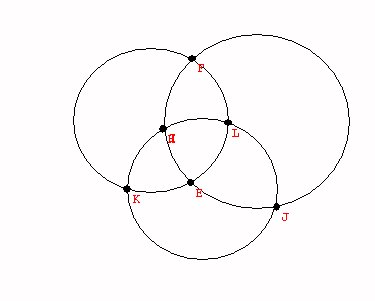Thursday,  March 4
Review of prvious discussion
What is the difference between Euclidean Geometry, Projective Geometry, and Topology

Euclid: congruence, similar... measurements, scale
Questions: Is a triangle congruent/similar to another triangle?
Is an circle congruent /similar to an ellipse?

Is a triangle congruent/similar to a square?

Projective: We will discuss this in greater detail later in the course.Projections preserve lines, points of intersection and contact (tangency).
Questions: Is a triangle projectively related to another triangle?
Is an circle projectively related to an ellipse?
Is a triangle
projectively related to a square?

• Topology vocabulary:

"Bounded" In the plane- A line segment is bounded. A line is not bounded. A circle is bounded.  A parabola is not bounded. "Bounded" means the object can be visualized in a box.

"
closed
"  A line segment with endpoints is closed. A circle is closed.  A line is closed. A line segment missing one or both endpoints in not closed. A circle missing one point is not closed.

"
open
"  A line segment missing both endpoints is open. A line is open.
A circle missing one point is open.

"
connected
" A line, a line segment, a circle, a circle missing one point,  and a parabola are all connected.
A line segment missing an interior point is not connected.
It has two pieces.

The following two  letters   are topologically equivalent.

I   C

The following two  letters   are topologically equivalent to each other but not to the previous two letters. This can be seen by removing a single point from these letters which will not disconnect the curves, as it does with the previous letters.

O  D

The letter T missing the point where the top meets the vertical line segment is not connected. It has three pieces.

The letter Y missing the point where the top meets the vertical line segment also is not connected. It has three pieces.
T and Y are topologically  the same (equivalent)! this can be seen by bending the tops to the letters up or down and stretching and shrinking the lengths as well.

Questions: Is a triangle topologically related to another triangle?
Yes. Stretch the sides and you'll also transform the angles.

Is an circle topologically related to a line?  No.
The circle is bounded and still connected when you remove a point.
The line is not bounded and is disconnected when you remove a point.

Is a triangle topologically related to a square?a circle? yes.

Topology and measurements:

• Counting on a line.  Counting on a curve.
Keep count on the number of vertices and segments for a graph.   Compare:

 Vertices Edges V-E Line segment I 2 1 1 circle O 1 1 0 8 1 2 -1 9 2 2 0 B 2 3 -1

Curves will not be topologically equivalent if the number V-E is not the same.

However, as the table shows, there are curves that have the same number V-E which are also not equivalent.

These can be distinguished by other criterion, such as connectivity when a point is removed.

The
number V-E is a characteristic of the curve
that will be the same for any topologically equivalent curve.

However, this number does not completely classify the curves topologically, since curves can have the same number V-E without being equivalent.

Counting in the plane or Counting on a surface.
Vertices, edges, regions.

 Vertices Regions Edges Line segment 2 1 1 circle 1 2 1 8 1 3 2 9 2 2 2 B 2 3 36 8 12

Counting in the plane or Counting on a sphere.

Euler's Formula for the plane or the sphere:
Theorem: For any connected graph in the plane,

V+R = E + 2.
OR  V -E+R =2

The number 2 is called the "Euler characteristic for the plane."  ( and the sphere).

Proof:  Deconstruct the graph.
First remove edges to "connect regions" until there is only one region.  The relation between V+R and E+ 2 stays the same.  (One edge goes with one region.)

Now remove one vertex and edge to "trim the tree".
Again the relation between V+R and E+2 stays the same. ( One edge goes with each vertex.)

Finally we have just one vertex, no edges, and one region.
So the relationship is that V+R = E + 2!

For any connected graph on the sphere, V+R = E + 2

Proof:
Choose a point P on the sphere not on the graph.
Place a plane touching the sphere opposite to P.
Then project the graph onto the plane.

The projected graph will have the same number of vertices, edges and regions.

The counting in the plane shows that the formula is true for the projected graph, and thus for the original graph on the sphere!

What about graphs on the Torus?
We have a connected graph on the torus with one vertex, two edges and only on region. But this information does not match for the euler formula for the plane or the sphere. SO.. the torus must be topologically different from the sphere or the plane!
In fact for the torus we see that it is possible for V+R= E   or  V-E+R= 0

Summary: We have learned about (and proved) Euler's Formula for the plane or the sphere:

For any connected graph in the plane or on the sphere, V+R = E + 2.
Next class we will see how this formula can help solve some problems on the plane and elsewhere.